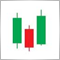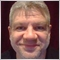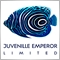# Convert indicator from Mt4 to Mt5.48

Hello everyone how are you, can someone help me convert this indicator from mt4 to mt5. Thank you very much....
```#property copyright ""
//----
#property indicator_chart_window
#property indicator_buffers 1
#property indicator_color1 Yellow
//----
extern int Snake_HalfCycle=5; // Snake_HalfCycle = 4...10 or other
//----
double Snake_Buffer[];
double Snake_Sum, Snake_Weight, Snake_Sum_Minus, Snake_Sum_Plus;
int Snake_FullCycle;
//----
int init()
{
int    draw_begin;
Snake_FullCycle=Snake_HalfCycle*2+1;
draw_begin=Snake_FullCycle+1;
SetIndexDrawBegin(0,draw_begin);
SetIndexBuffer(0,Snake_Buffer);
SetIndexStyle(0,DRAW_LINE);
return(0);
}
//----
int start()
{
int FirstPos, ExtCountedBars=0;
if(Bars<=150) return(0);
if(Snake_HalfCycle<3) Snake_HalfCycle=3;
ExtCountedBars=IndicatorCounted();
if (ExtCountedBars<0) return(-1);
if (ExtCountedBars>0) ExtCountedBars--;
FirstPos=Bars-ExtCountedBars-1;
if(FirstPos>Bars-Snake_HalfCycle-1) FirstPos=Bars-Snake_HalfCycle-1;
Snake(FirstPos);
return(0);
}
//----
void Snake(int Pos)
{
int i;
if(Pos<=Snake_HalfCycle+1) Pos=Snake_HalfCycle+2;
Snake_Buffer[Pos]=SnakeFirstCalc(Pos);
Pos--;
while(Pos>=Snake_HalfCycle)
{
Snake_Buffer[Pos]=SnakeNextCalc(Pos);
Pos--;
}
while(Pos>0)
{
Snake_Buffer[Pos]=SnakeFirstCalc(Pos);
Pos--;
}
if(Pos==0)
Snake_Buffer=iMA(NULL,0,Snake_HalfCycle,0,MODE_LWMA,PRICE_TYPICAL,0);
return;
}
//----
double SnakePrice(int Shift)
{
return((2*Close[Shift]+High[Shift]+Low[Shift])/4);
}
//----
double SnakeFirstCalc(int Shift)
{
int i, j, w;
Snake_Sum=0.0;
Snake_Weight=0.0;
if(Shift<Snake_HalfCycle)
{
i=0;
w=Shift+Snake_HalfCycle;
while(w>=Shift)
{
i++;
Snake_Sum=Snake_Sum+i*SnakePrice(w);
Snake_Weight=Snake_Weight+i;
w--;
}
while(w>=0)
{
i--;
Snake_Sum=Snake_Sum+i*SnakePrice(w);
Snake_Weight=Snake_Weight+i;
w--;
}
}
else
{
Snake_Sum_Minus=0.0;
Snake_Sum_Plus=0.0;
for(j=Shift-Snake_HalfCycle,i=Shift+Snake_HalfCycle,w=1;
w<= Snake_HalfCycle;
j++,i--,w++)
{
Snake_Sum=Snake_Sum+w*(SnakePrice(i)+SnakePrice(j));
Snake_Weight=Snake_Weight+2*w;
Snake_Sum_Minus=Snake_Sum_Minus+SnakePrice(i);
Snake_Sum_Plus=Snake_Sum_Plus+SnakePrice(j);
}
Snake_Sum=Snake_Sum+( Snake_HalfCycle+1)*SnakePrice(Shift);
Snake_Weight=Snake_Weight+ Snake_HalfCycle+1;
Snake_Sum_Minus=Snake_Sum_Minus+SnakePrice(Shift);
}
return(Snake_Sum/ Snake_Weight);
}
//----
double SnakeNextCalc(int Shift)
{
Snake_Sum_Plus=Snake_Sum_Plus+SnakePrice(Shift-Snake_HalfCycle);
Snake_Sum=Snake_Sum-Snake_Sum_Minus+Snake_Sum_Plus;
Snake_Sum_Minus=Snake_Sum_Minus-SnakePrice(Shift+Snake_HalfCycle+1)+SnakePrice(Shift);
Snake_Sum_Plus=Snake_Sum_Plus-SnakePrice(Shift);
return(Snake_Sum/Snake_Weight);
}
//----

```
Files:
Snake.mq4  4 kb42187

In my opinion, help means to support and provide answers when someone doesn't understand something or when he is stuck while trying to do something.

What have you tried or what don't you understand about converting mql4 indicator to mql5 ?25319

Help you with what? You haven't stated a problem, you stated a want. You have only four choices:
1. Search for it.

2. Beg at

3. MT4: Learn to code it.
MT5:
If you don't learn MQL4/5, there is no common language for us to communicate. If we tell you what you need, you can't code it. If we give you the code, you don't know how to integrate it into yours.

4. or pay (Freelance) someone to code it.
Hiring to write script - General - MQL5 programming forum

We're not going to code it for you (although it could happen if you are lucky or the problem is interesting.) We are willing to help you when you post your attempt (using CODE button) and state the nature of your problem.
No free help48

Alain Verleyen:

In my opinion, help means to support and provide answers when someone doesn't understand something or when he is stuck while trying to do something.

What have you tried or what don't you understand about converting mql4 indicator to mql5 ?

I just started trading recently so I don't have much experience. how can I use a mt4 indecator on the mt5 platform. communicate here thanks for the reply48

William Roeder:
Help you with what? You haven't stated a problem, you stated a want. You have only four choices:
1. Search for it.

2. Beg at

3. MT4: Learn to code it.
MT5:
If you don't learn MQL4/5, there is no common language for us to communicate. If we tell you what you need, you can't code it. If we give you the code, you don't know how to integrate it into yours.

4. or pay (Freelance) someone to code it.
Hiring to write script - General - MQL5 programming forum

We're not going to code it for you (although it could happen if you are lucky or the problem is interesting.) We are willing to help you when you post your attempt (using CODE button) and state the nature of your problem.
No free help

I just started trading recently so I don't have much experience. thanks for the replyModerator
17991

chokri77:

I just started trading recently so I don't have much experience. how can I use a mt4 indecator on the mt5 platform. communicate here thanks for the reply

You can't use a MT4 indicator in MT5 platform.48

HI Everyone,I try to convert this indicator mql4 to mql5 but every time give me the some error . see the picture below for more understand, someone can help me out with this problem. Thank you in advance.

```//---- version
#property version   "1.00"
//---- draw indicator in a separated window
#property indicator_chart_window
//---- one indicator buffer is used
#property indicator_buffers 5
//---- one graphic plot is used
#property indicator_plots   1
//+-----------------------------------+
//|  Indicator plot settings          |
//+-----------------------------------+
//---- draw as a line
#property indicator_type1   DRAW_LINE
//---- line color (Red)
#property indicator_color1 clrYellow
//---- line style (solid line)
#property indicator_style1  STYLE_SOLID
//---- line width
#property indicator_width1  2
//---- line label
#property indicator_label1  "SNAKE"

ENUM_TIMEFRAMES TFMigrate(int tf)
{
switch(tf)
{
case 0: return(PERIOD_CURRENT);
case 1: return(PERIOD_M1);
case 5: return(PERIOD_M5);
case 15: return(PERIOD_M15);
case 30: return(PERIOD_M30);
case 60: return(PERIOD_H1);
case 240: return(PERIOD_H4);
case 1440: return(PERIOD_D1);
case 10080: return(PERIOD_W1);
case 43200: return(PERIOD_MN1);

case 2: return(PERIOD_M2);
case 3: return(PERIOD_M3);
case 4: return(PERIOD_M4);
case 6: return(PERIOD_M6);
case 10: return(PERIOD_M10);
case 12: return(PERIOD_M12);
case 16385: return(PERIOD_H1);
case 16386: return(PERIOD_H2);
case 16387: return(PERIOD_H3);
case 16388: return(PERIOD_H4);
case 16390: return(PERIOD_H6);
case 16392: return(PERIOD_H8);
case 16396: return(PERIOD_H12);
case 16408: return(PERIOD_D1);
case 32769: return(PERIOD_W1);
case 49153: return(PERIOD_MN1);
default: return(PERIOD_CURRENT);
}
}

//---- indicator inputs
input int Snake_HalfCycle=5; // Snake_HalfCycle = 4...10 or other

double Snake_Sum, Snake_Weight, Snake_Sum_Minus, Snake_Sum_Plus;
int Snake_FullCycle;

//+-----------------------------------+
//---- indicator buffers
double Ind_Buffer[];

//----
double dPriceShift;
//+------------------------------------------------------------------+
// CT3 class, iPriceSeries(),iPriceSeriesAlert() functions are used  |
//+------------------------------------------------------------------+

//+------------------------------------------------------------------+
//| T3 indicator initialization function                             |
//+------------------------------------------------------------------+
void OnInit()
{
int    draw_begin;
Snake_FullCycle=Snake_HalfCycle*2+1;
draw_begin=Snake_FullCycle+1;

//---- set Ind_Buffer[] array as an indicator buffer
SetIndexBuffer(0,Ind_Buffer,INDICATOR_DATA);
//---- set plot shift (horizontal shift in bars)
PlotIndexSetInteger(0,PLOT_DRAW_BEGIN,draw_begin);
//--- indicator label, shown in the DataWindow
PlotIndexSetString(0,PLOT_LABEL,"SNAKE");
//---- set empty values
PlotIndexSetDouble(0,PLOT_EMPTY_VALUE,EMPTY_VALUE);
//---- variable for indicator short name
//---- set indicator short name
IndicatorSetString(INDICATOR_SHORTNAME,"SNAKE");
//---- set precision
IndicatorSetInteger(INDICATOR_DIGITS,_Digits+1);
//---- initialization end

}
//+------------------------------------------------------------------+
//| T3 iteration function                                            |
//+------------------------------------------------------------------+
int OnCalculate(const int rates_total,
// rates total
const int prev_calculated,// number of bars, calculated at previous call
const datetime &time[],
const double &open[],
const double &high[],
const double &low[],
const double &close[],
const long &tick_volume[],
const long &volume[],
)
{

//---- bars checking
if(rates_total<0)return(0);

//---- declaration of variables of integer type
int first;

//---- calculation of starting bar index (first)
if(prev_calculated>rates_total || prev_calculated<=0) // at first call
first=0; // starting bar index
else first=prev_calculated-1; // starting bar index for new bars

int Pos = first;

int bars=Bars(_Symbol,_Period);

if(Pos>bars-Snake_HalfCycle-1)
Pos=bars-Snake_HalfCycle-1;

int      buffer_num=0;           // indicator buffer number
int      start_pos=0;            // start position
int      count=500;                // amount to copy

/*  SNAKE bar  */

if(Pos<=Snake_HalfCycle+1)
{
Pos=Snake_HalfCycle+2;

}

Ind_Buffer[Pos]=SnakeFirstCalc(Pos,open,high,low,close);
Pos--;

while(Pos>=Snake_HalfCycle)
{
Ind_Buffer[Pos]=SnakeNextCalc(Pos,open,high,low,close);
Pos--;

}
while(Pos>0)
{
Ind_Buffer[Pos]=SnakeFirstCalc(Pos,open,high,low,close);
Pos--;

}
if(Pos==0)
Ind_Buffer=iMA(NULL,0,Snake_HalfCycle,0,MODE_LWMA,PRICE_TYPICAL);

return(rates_total);
}
//+------------------------------------------------------------------+

//----
double SnakePrice(int Shift,
const double &open[],
const double &high[],
const double &low[],
const double &close[])
{

return((2*close[Shift]+high[Shift]+low[Shift])/4);
}
//----
double SnakeFirstCalc(int Shift,const double &open[],
const double &high[],
const double &low[],
const double &close[])
{
int i, j, w;
Snake_Sum=0.0;
Snake_Weight=0.0;
if(Shift<Snake_HalfCycle)
{
i=0;
w=Shift+Snake_HalfCycle;
while(w>=Shift)
{
i++;
Snake_Sum=Snake_Sum+i*SnakePrice(w,open,high,low,close);
Snake_Weight=Snake_Weight+i;
w--;
}
while(w>=0)
{
i--;
Snake_Sum=Snake_Sum+i*SnakePrice(w,open,high,low,close);
Snake_Weight=Snake_Weight+i;
w--;
}
}
else
{
Snake_Sum_Minus=0.0;
Snake_Sum_Plus=0.0;
for(j=Shift-Snake_HalfCycle,i=Shift+Snake_HalfCycle,w=1;
w<= Snake_HalfCycle;
j++,i--,w++)
{
Snake_Sum=Snake_Sum+w*(SnakePrice(i,open,high,low,close)+SnakePrice(j,open,high,low,close));
Snake_Weight=Snake_Weight+2*w;
Snake_Sum_Minus=Snake_Sum_Minus+SnakePrice(i,open,high,low,close);
Snake_Sum_Plus=Snake_Sum_Plus+SnakePrice(j,open,high,low,close);
}
Snake_Sum=Snake_Sum+( Snake_HalfCycle+1)*SnakePrice(Shift,open,high,low,close);
Snake_Weight=Snake_Weight+ Snake_HalfCycle+1;
Snake_Sum_Minus=Snake_Sum_Minus+SnakePrice(Shift,open,high,low,close);
}
return(Snake_Sum/ Snake_Weight);
}
//----
double SnakeNextCalc(int Shift,
const double &open[],
const double &high[],
const double &low[],
const double &close[])
{
Snake_Sum_Plus=Snake_Sum_Plus+SnakePrice(Shift-Snake_HalfCycle,open,high,low,close);
Snake_Sum=Snake_Sum-Snake_Sum_Minus+Snake_Sum_Plus;
Snake_Sum_Minus=Snake_Sum_Minus-SnakePrice(Shift+Snake_HalfCycle+1,open,high,low,close)+SnakePrice(Shift,open,high,low,close);
Snake_Sum_Plus=Snake_Sum_Plus-SnakePrice(Shift,open,high,low,close);
return(Snake_Sum/Snake_Weight);
}
//----

```
Files:
SNAKER.ex5  21 kb42187

chokri77:

HI Everyone,I try to convert this indicator mql4 to mql5 but every time give me the some error . see the picture below for more understand, someone can help me out with this problem. Thank you in advance.

Your indexing is not correct. By default MT5 doesn't set arrays/buffers indexed as series. So the oldest value is a index 0 and the most recent at rates_total-1.48

Alain Verleyen:
Your indexing is not correct. By default MT5 doesn't set arrays/buffers indexed as series. So the oldest value is a index 0 and the most recent at rates_total-1.

HI Alain Verleyen, Thank you for your help. but I didn't understand which index to change. I followed a guide that explains how to convert step by step . thank you42187

chokri77:

HI Alain Verleyen, Thank you for your help. but I didn't understand which index to change. I followed a guide that explains how to convert step by step . thank you

Sorry but I don't have time for that.```   ArraySetAsSeries(time,true);